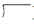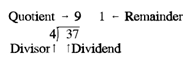## Dividing Whole Numbers Assignment Help

Assignment Help: >> Basic Arithmetic Operations - Dividing Whole Numbers

Dividing Whole Numbers:

Division is the procedure of determining how several times one number is contained in another number.  While numbers are divided, the result is the quotient and a remainder.  The remainder is what remains after division.  The number divided by another number is known as the dividend; the number divided into the dividend is known as the divisor.  Division is implies by any of the following:

a division sign (÷)

a division sign ()

a horizontal line along with the dividend above the line and the divisor below the line       #/#

the slanting line a/b  meaning  a divided by b

Therefore, the relationship among the dividend, divisor, and quotient is as display below:

37        Dividend

÷ 4       Divisor

----------------------

9          Quotient

1          RemainderUnlike multiplication, the division procedure is neither associative nor commutative. The commutative law for multiplication allows reversing the order of the factors without changing the product.  In the division process the dividend and divisor cannot be reversed.

Using the equation form:

a ÷ b ≠ b ÷ a

For example, the quotient of 18 ÷ 6 is not the similar as the quotient of 6 ÷ 18.  18 divided by 6 equals 3; 6 divided by 18 equals 0.33.